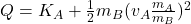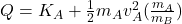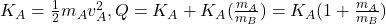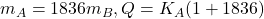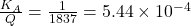## A neutron at rest decays (breaks up) to a proton and an electron. Energy is released in the decay and appears as kinetic energy of the proto

Question

A neutron at rest decays (breaks up) to a proton and an electron. Energy is released in the decay and appears as kinetic energy of the proton and electron. The mass of a proton is 1836 times the mass of an electron. The mass of a proton is 1836 times the mass of an electron. What fraction of the total energy released goes into the kinetic energy of the proton?

in progress 0
5 months 2021-08-13T09:54:27+00:00 1 Answers 481 views 0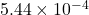Explanation:

Let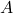and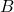represent proton and electron respectively

Total energy decay is: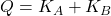(1)

where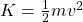(2)

The momentum of the particle is given by: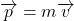After the decay we have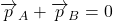(3)

Since the particles move in opposite direction,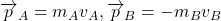From eq (3) we get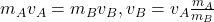(4)

From eq (2) we get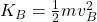From eq (1) and (4),